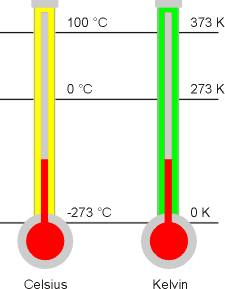# What do you mean by Thermodynamic scale?

Most of the temperature scales are based on the nature and thermometric properties of working substances. The use of different thermometric substances and their properties make the scales different. Even, the thermal expansion or contraction of a thermometric substance may not vary uniformly in all temperature regions. So, a single value of temperature is different in different scale. To overcome these difficulties Lord Kelvin, in 1848 introduced a scale of temperature, which does not depend on the nature and properties of the thermometric substance, but based on the temperature only (i.e. depends on the working principle of Carnot’s engine). This scale is known as Kelvin scale or Thermodynamic scale.

As this scale is independent of the nature and properties of the thermometric substance, it is called absolute scale of temperature.

The temperature at which the pressure (P) and volume (V) becomes zero is called lowest temperature -273oC or 0K this is called the absolute zero temperature.

• 20oC = 273 + 20 = 293K and -32oC = 273 – 32 = 241KThermodynamic scale

This scale is found to agree exactly with the perfect gas scale. The temperature T in perfect gas scale or in thermodynamic scale is obtained as: T = 273.16 PT/Ptr

Here, PT = Pressure of ideal gas at temperature T Kelvin

Ptr = Pressure of Ideal gas of the same volume at the triple point of water.